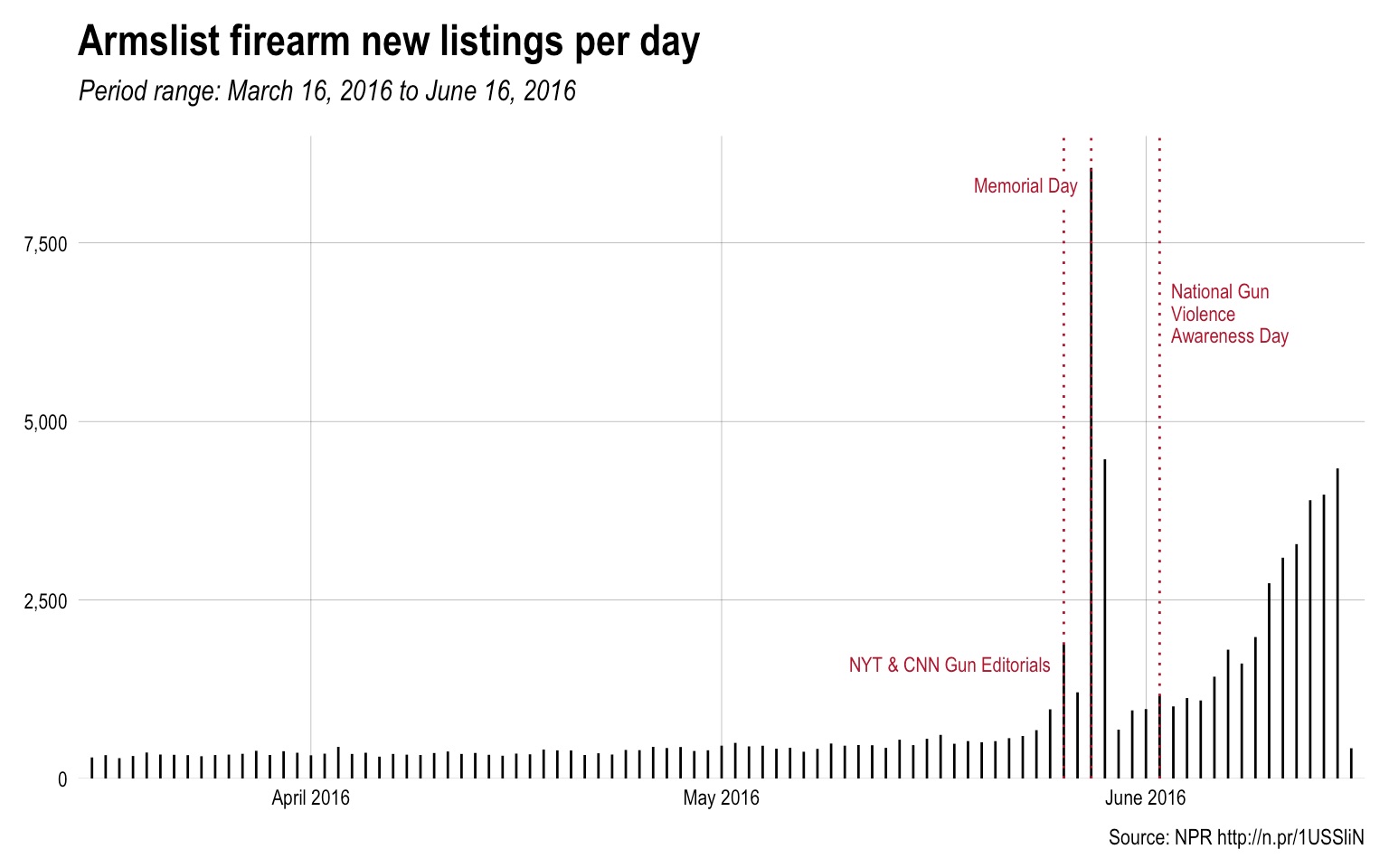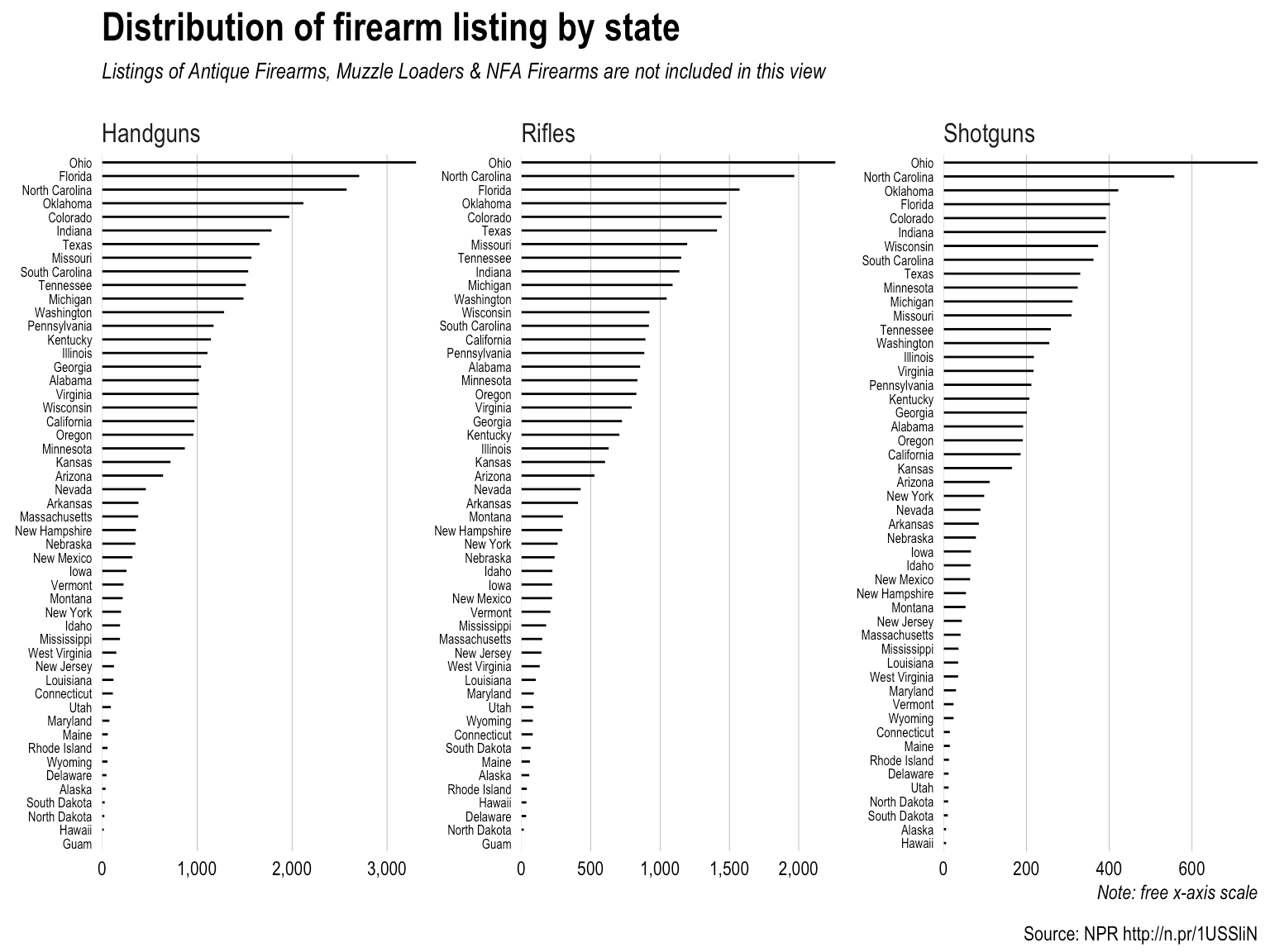# A Call to Arms[list] Data Analysis!

The NPR vis team contributed to a recent [story](http://n.pr/1USSliN) about Armslist, a “craigslist for guns”. Now, I’m neither pro-“gun” or anti-“gun” since this subject, like most heated ones, has more than two sides. What I _am_ is pro-*data*, and the U.S. Congress is so [deep in the pockets of the NRA](http://abcnews.go.com/Health/cdc-launched-comprehensive-gun-study-15-years/story?id=39873289) that there’s no way for there to be any Federally-supported, data-driven research on gun injuries/deaths. Thankfully, California is going to [start funding research](http://www.wired.com/2016/06/congress-refuses-california-funds-gun-violence-research-center/), so we may see some evidence-based papers in the (hopefully) not-too-distant future.

When I read the NPR story I couldn’t believe it was easier to get a gun than it is get [pick your vice or other bit of dangerous contraband]. The team at NPR ended up [scraping the Armslist site](http://blog.apps.npr.org/2016/06/17/scraping-tips.html) and provided a [CSV of the data](http://apps.npr.org/armslist-analysis/armslist-listings-2016-06-16.csv). Their own blog post admirably started off with a “Can you scrape?” section. This is an area I see so many python, R and other folks totally ignore since they seem to feel that just because you _can_ do something also gives you license to do so.

I’m glad the NPR team provided the CSV of their results since I suspect that Armslist will be adding some “no scraping” language to their Terms of Service. Interestingly enough, the Armslist site owners spend a great deal of verbiage across their site indemnifying themselves (that’s how proud of their service they are).

Since they provided the CSV, I poked at it a bit and produced some alternate views of the data. One bit of info I was interested in is how much the ask price was for the firearms. Since this is a craigslist-like site, some of the prices are missing and others are obviously either “filler” like `12345678` or are legitimately large (i.e. the price for a rare antique). Given the huge right-skew, I limited the initial view to “affordable” ones (which I defined as between \$0.00 & \$2,500 USD and if you look at the data yourself you’ll see why). I then computed the bandwidth for the density estimate and did some other basic maths to see what price range of the offers made up at least 50% of the overall listings. I probably should have excluded the \$1 offers but the data is there for you to use to augment anything I’ve done here.Most of these firearms are quite affordable (even if you ignore the \$1.00 USD offers).

One other view I wanted to see was that of the listings-per-day.Info from the NPR vis team suggests this is not a 100% accurate view since the listings “age out” and they did a point-in-time scrape. It would be interesting to start a daily scraper for this site or ask to work with the raw data from the site itself (but it’s unlikely Armslist would have the courage to make said data available to news organizations or researchers). Also, the value for the last segment-bar does not appear to be from a fully day’s scrape. Nothing says ‘Murica like selling guns in a sketchy way for Memorial Day.

Finally, I wanted a different view of the ranked states.(The `ggplot2` code for this one is kinda interesting for any R folk who are curious). This segment-bar chart is a bit of an eye strain (click on it to make it larger) but the main thing I wanted to see was if Ohio was as gun-nutty for the three less-than-valid (IMO) types of firearms sales (which is a different view than automatic vs semi-automatic). Sure enough, Ohio leads the pack (in other news, the same states are in the top 5 across these three categories).

“Spinnable” R code for these charts is below, so go forth and see if you can tease out any other views from the data. There is a free-text listing description field which may be interesting to mine, and the R code has sorted lists by manufacturer and caliber you can view if you run/spin it. It might be interesting to get data [like this for Ohio](https://en.wikipedia.org/wiki/Gun_laws_in_Ohio) for other states and do some clustering based on the legal categories outlined in the table.

``````#' ---
#' output:
#'   html_document:
#'     keep_md: true
#' ---

#+ message=FALSE, echo=FALSE, warning=FALSE
library(dplyr)
library(ggalt)
library(hrbrmisc)
library(KernSmooth)
library(scales)
library(stringi)
library(extrafont)
library(DT)

arms <- mutate(arms,
price=ifelse(price=="FREE", 0, price),
price=ifelse(price=="Offer", NA, price),
price=make_numeric(price))
arms <- mutate(arms,
listed_date=gsub("^.*y, ", "", listed_date),
listed_date=as.Date(listed_date, "%B %d, %Y"))

affordable <- filter(arms, price>0 & price<2500)

bw <- dpik(affordable\$price, scalest="stdev")

a_dens <- bkde(affordable\$price, bandwidth=bw,
range.x=range(affordable\$price),
truncate=TRUE)

peaks <- data_frame(
pk=which(diff(sign(diff(c(0, a_dens\$y)))) == -2),
x=a_dens\$x[pk],
y=a_dens\$y[pk]
)

ann <- sprintf('%s (%s of all listings) firearms are\noffered between \$1 & \$600 USD',
comma(nrow(filter(affordable, between(price, 1, 600)))),
percent(nrow(filter(affordable, between(price, 1, 600)))/nrow(arms)))

grps <- setNames(1:6, unique(arms\$category))

ggplot() +
geom_segment(data=cbind.data.frame(a_dens), aes(x, xend=x, 0, yend=y),
color="#2b2b2b", size=0.15) +
geom_vline(data=peaks[c(1,8),], aes(xintercept=x), size=0.5,
linetype="dotted", color="#b2182b") +
geom_label(data=peaks[c(1,8),], label.size=0,
aes(x, y, label=dollar(floor(x)), hjust=c(0, 0)),
nudge_x=c(10, 10), vjust=0, size=3,
family="Arial Narrow") +
geom_label(data=data.frame(), hjust=0, label.size=0, size=3,
aes(label=ann, x=800, y=min(a_dens\$y) + sum(range(a_dens\$y))*0.7),
family="Arial Narrow") +
scale_x_continuous(expand=c(0,0), breaks=seq(0, 2500, 500), label=dollar, limits=c(0, 2500)) +
scale_y_continuous(expand=c(0,0), limits=c(0, max(a_dens\$y*1.05))) +
labs(x=NULL, y="density",
title="Distribution of firearm ask prices on Armslist",
subtitle=sprintf("Counts are across all firearm types (%s)",
stri_replace_last_regex(paste0(names(grps), collapse=", "), ",", " &")),
caption="Source: NPR http://n.pr/1USSliN") +
theme_hrbrmstr_an(grid="X=Y", subtitle_size=10) +
theme(axis.text.x=element_text(hjust=c(0, rep(0.5, 4), 1))) +
theme(axis.text.y=element_blank()) +
theme(plot.margin=margin(10,10,10,10)) -> gg

#+ ask-prices, dev="png", fig.width=8, fig.height=4, fig.retina=2, message=FALSE, echo=FALSE, warning=FALSE
gg

count(arms, state, category) %>%
group_by(category) %>%
mutate(f=paste0(paste0(rep(" ", grps[category]), collapse=""), state, collaspe="")) %>%
ungroup() %>%
arrange(desc(n)) %>%
mutate(f=factor(f, levels=rev(f))) %>%
filter(category %in% c("Handguns", "Rifles", "Shotguns")) %>%
ggplot(aes(x=n, y=f)) +
geom_segment(aes(yend=f, xend=0), size=0.5) +
scale_x_continuous(expand=c(0,0), label=comma) +
facet_wrap(~category, scales="free") +
labs(x="Note: free x-axis scale", y=NULL,
title="Distribution of firearm listing by state",
subtitle="Listings of Antique Firearms, Muzzle Loaders & NFA Firearms are not included in this view",
caption="Source: NPR http://n.pr/1USSliN") +
theme_hrbrmstr_an(grid="X", subtitle_size=10) +
theme(axis.text.y=element_text(size=6)) -> gg

#+ by-state, dev="png", fig.width=8, fig.height=6, fig.retina=2, message=FALSE, echo=FALSE, warning=FALSE
gg

count(arms, listed_date) %>%
ggplot(aes(listed_date, n)) +
geom_segment(aes(xend=listed_date, yend=0)) +
geom_vline(xintercept=c(as.numeric(c(as.Date("2016-05-26"),
as.Date("2016-05-28"),
as.Date("2016-06-02")))), color="#b2182b", size=0.5, linetype="dotted") +
geom_label(data=data.frame(), hjust=1, vjust=1, nudge_x=-0.5, label.size=0, size=3,
aes(x=as.Date("2016-05-26"), y=1800, label="NYT & CNN Gun Editorials"),
family="Arial Narrow", color="#b2182b") +
geom_label(data=data.frame(), hjust=1, vjust=1, nudge_x=-0.5, label.size=0, size=3,
aes(x=as.Date("2016-05-28"), y=8500, label="Memorial Day"),
family="Arial Narrow", color="#b2182b") +
geom_label(data=data.frame(), hjust=0, vjust=1, nudge_x=0.5,
label.size=0, size=3, lineheight=0.9,
aes(x=as.Date("2016-06-02"), y=7000,
label="National Gun\nViolence\nAwareness Day"),
family="Arial Narrow", color="#b2182b") +
scale_x_date(expand=c(0,1), label=date_format("%B 2016")) +
scale_y_continuous(expand=c(0,0), label=comma, limit=c(0, 9000)) +
labs(x=NULL, y=NULL,
title="Armslist firearm new listings per day",
subtitle="Period range: March 16, 2016 to June 16, 2016",
caption="Source: NPR http://n.pr/1USSliN") +
theme_hrbrmstr_an(grid="XY") +
theme(plot.margin=margin(10,10,10,10)) -> gg

#+ per-day, dev="png", fig.width=8, fig.height=5, fig.retina=2, message=FALSE, echo=FALSE, warning=FALSE
gg

count(arms, manufacturer) %>%
filter(!is.na(manufacturer)) %>%
arrange(desc(n)) %>%
select(Manufacturer=manufacturer, Count=n) %>%
datatable() %>%
formatCurrency(columns="Count", currency="")

count(arms, caliber) %>%
filter(!is.na(caliber)) %>%
arrange(desc(n)) %>%
select(Caliber=caliber, Count=n) %>%
datatable() %>%
formatCurrency(columns="Count", currency="")``````Amazon Author Page

## 3 Comments →A Call to Arms[list] Data Analysis!

1. Pingback: Linkdump #2 | WZB Data Science Blog

This site uses Akismet to reduce spam. Learn how your comment data is processed.